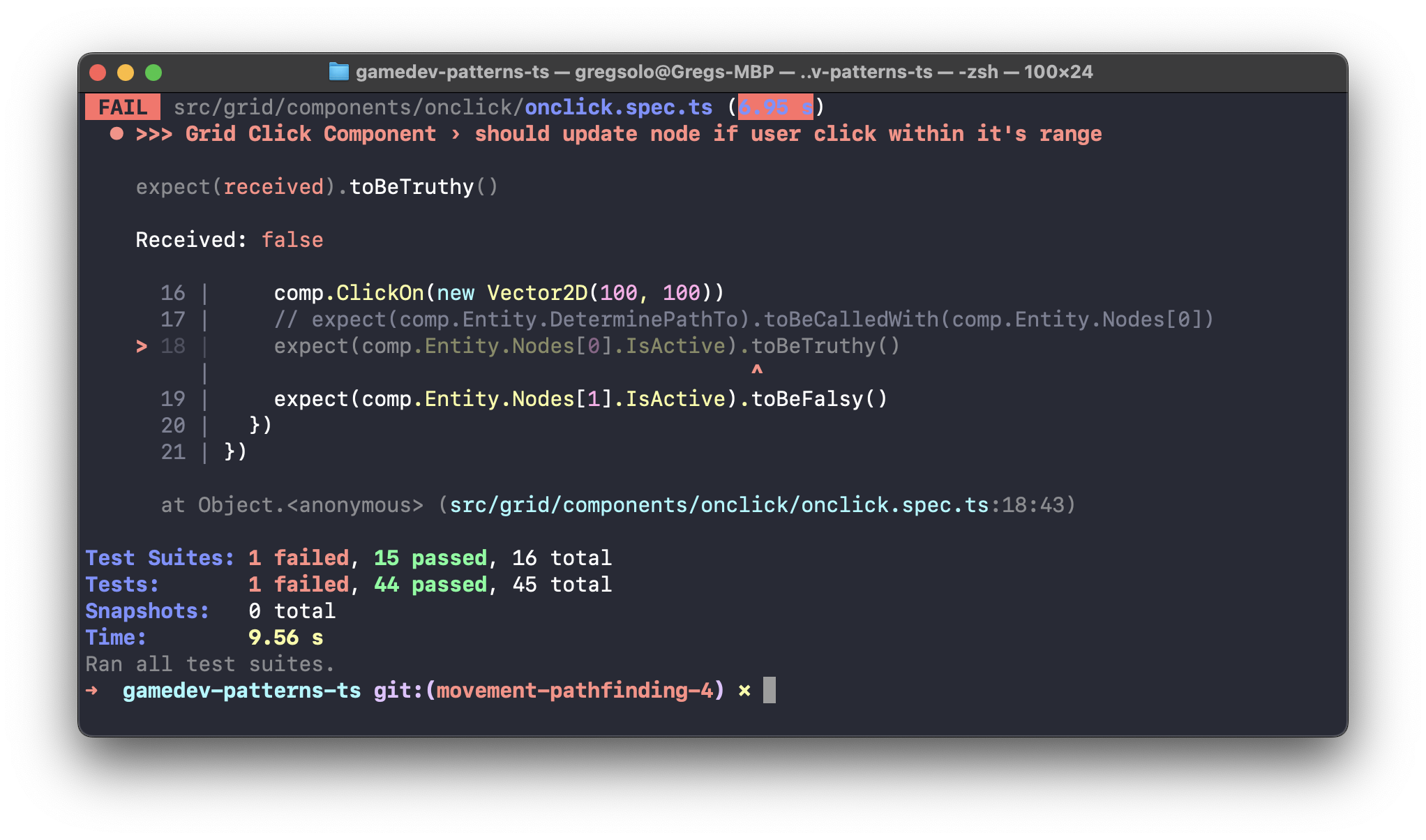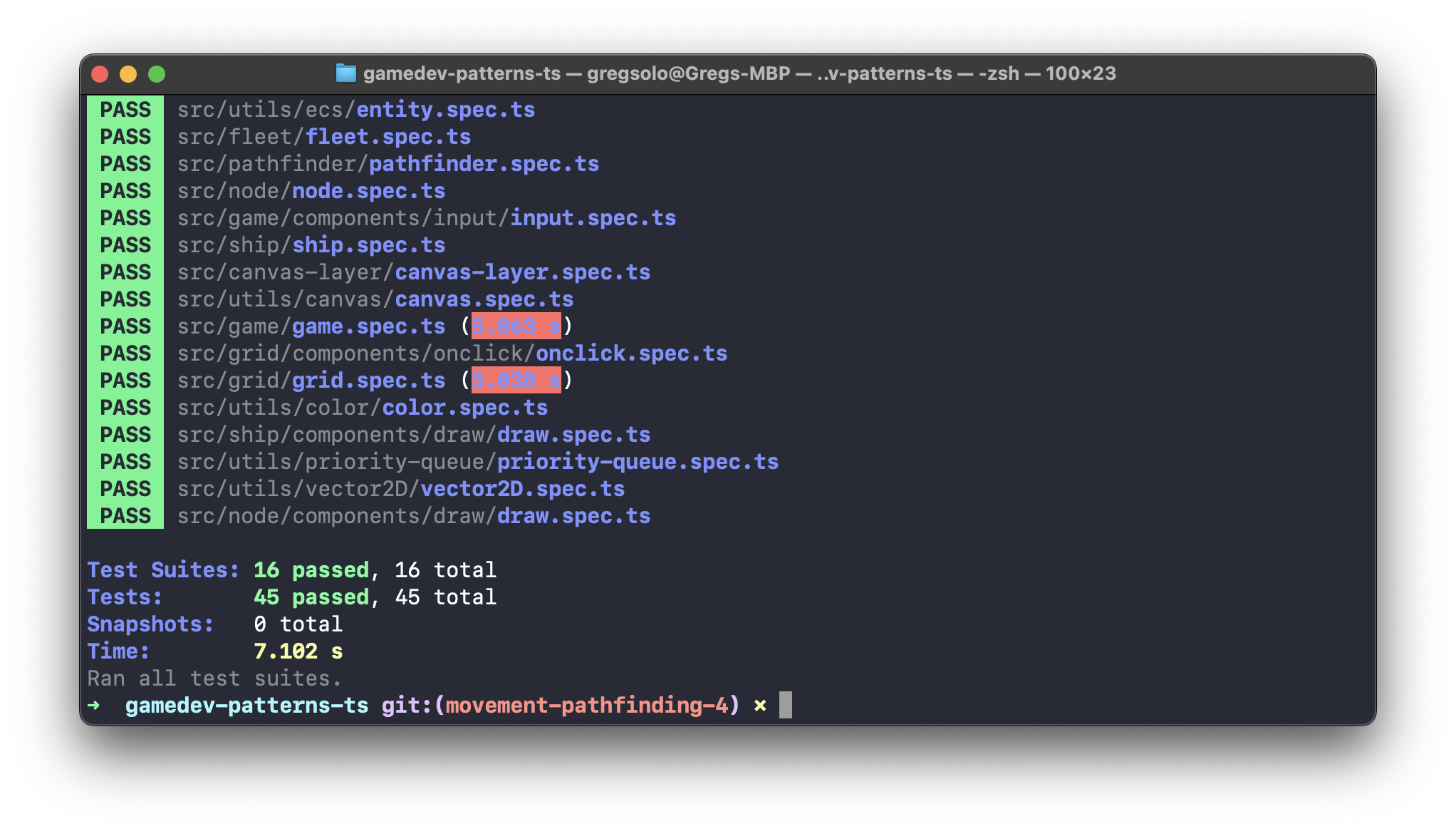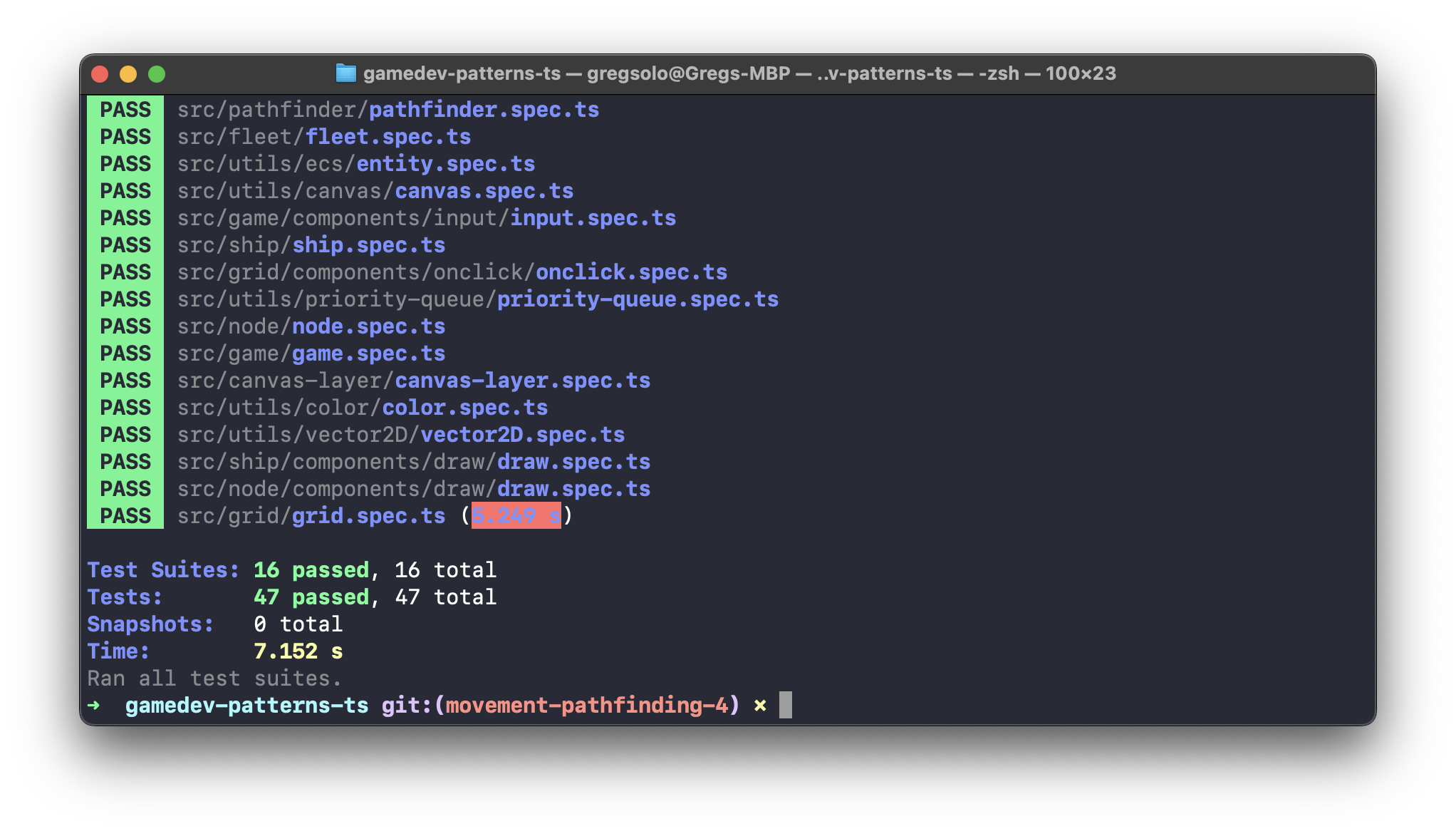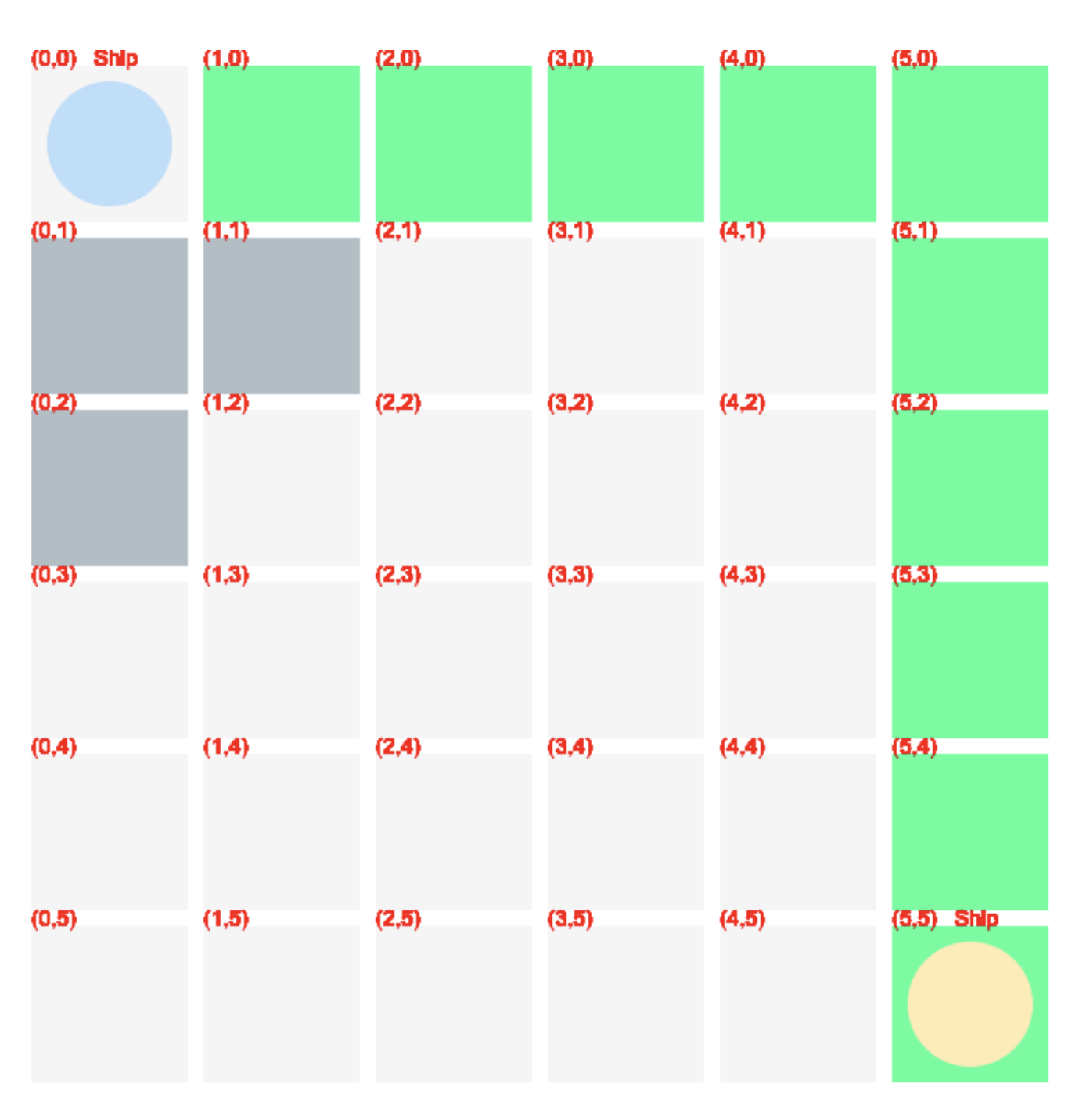# Building a Game With TypeScript. Pathfinding and Movement 5/7. Finding the Path

Chapter VI in the series of tutorials on how to build a game from scratch with TypeScript and native browser APIsCute astronaut vector created by catalyststuff - www.freepik.com

Hello there! I hope you are enjoying this series so far. Last time we successfully prepared our Pathfinder that helps calculate the path on some abstract `Graph`. In this installment, we are going to use it and build a path on our real `Grid`

In Chapter VI “Pathfinding and Movement ”, we finally make our Ships alive! You can find other Chapters of this series here:

Feel free to switch to the `movement-pathfinding-4` branch of the repository. It contains the working result of the previous posts and is a great starting point for this one.

1. Introduction
2. Grid as a Graph
3. Visualizing the Path
4. Testing OnClick and Draw components
5. Testing DeterminePathTo
6. Conclusion

## Grid as a Graph

Our Pathfinder relies on `IGraph` for its calculations. Simply put, we need someone to implement the `IGraph` we created in the last installment. And there is no better candidate than `Grid` since it handles all bulk logic around `Node:

``````// src/grid/grid.ts
import { Entity, Vector2D, IGraph, IGraphNode } from '@/utils' // <--- CHANGE
// ... //

export class Grid extends Entity implements IGraph { // <--- CHANGE

// ... //

public GetCost(a: IGraphNode, b: IGraphNode): number {
throw new Error('Method not implemented.')
}
public GetNeighborsOf(node: IGraphNode): IGraphNode[] {
throw new Error('Method not implemented.')
}

private InitNodes(): void {
// ... //
}
}
``````

And we can also ask `Node` to conform to `IGraphNode`:

``````// src/node/node.ts
// ... //
import { Entity, Vector2D, IGraphNode } from '@/utils' // <--- CHANGE
// ... //

export class Node extends Entity implements IGraphNode { // <--- CHANGE
// ... //
public get Position(): Vector2D {
return this.Index
}
constructor(...){
// ... //
}
// ... //
}

``````

All we need is to implement a `Position` getter which is incredibly easy for us since we already have something that can be seen as "Position" on the graph: `Index`!

We probably could even use `Center` for this purpose but it has additional complexity: physical `canvas` coordinates of the center of the `Node` calculated based on the start position and size. We don't need this complication, the `Index` that defines position within the grid coordinate system is all we need.

Having `Node` that conforms to `IGraphNode`, we can now reference it instead of the abstract `IGraphNode` interface:

``````// src/grid/grid.ts
// ... //
export class Grid extends Entity implements IGraph {
// ... //
public GetCost(a: Node, b: Node): number { // <--- CHANGE
return 1 // <--- CHANGE
}

public GetNeighborsOf(node: Node): Node[] { // <--- CHANGE
return node.Neighbors // <--- CHANGE
}
// ... //
}
``````

Note that I also edited the methods to make them do some real jobs. `GetNeighborsOf` simply returns `Neighbors` of the `Node` while `GetCost` always returns 1, meaning that all Nodes are equally accessible on our grid.

Now, let's introduce a pathfinder and ask it to calculate a path for us:

``````// src/grid/grid.ts
// ... //
import { Pathfinder } from '@/pathfinder' // <--- ADD
import { GridOnclickComponent } from './components'

export class Grid extends Entity implements IGraph {
private _nodes: Node[] = []
private _pathfinder = new Pathfinder(this, Grid.Heuristic) // <--- ADD
private _currentPath: Node[] = [] // <--- ADD

public static Heuristic = (a: IGraphNode, b: IGraphNode): number => Math.abs(a.Position.x - b.Position.x) + Math.abs(a.Position.y - b.Position.y) // <--- ADD

// ... //
public GetNeighborsOf(node: Node): Node[] {
return node.Neighbors
}

public DeterminePathTo(node: Node): void {}
// ... //
}
``````

A few things happen here. First, we define a private field `_pathfinder` and instantiate `Pathfinder` into it. It requires 2 things to be instantiated as well: graph (which is our `Grid`) and `heuristic` function. For the latter I defined a static property `Heuristic` that returns Manhattan distance between 2 nodes. That's more than enough at this point.

I also stored `_currentPath` which will be calculated when `DeterminePathTo(node: Node)` is called.

Well, what are we waiting for? Let's go ahead and indeed call it:

``````// src/grid/components/onclick/onclick.ts
// ... //
export class GridOnclickComponent extends OnclickComponent {
public ClickOn(point: Vector2D): void {
for (const node of this.Entity.Nodes) {
node.IsActive = node.Occupies(point) // <--- REMOVE
if (node.Occupies(point)) {
this.Entity.DeterminePathTo(node)
}
}
}
}
``````

Do you remember what `ClickOn` does? It gets invoked when we click anywhere on the grid. `node.Occupies(point)` helps us determine which node exactly is under click and we can supply it to `DeterminePathTo`. Easy!

Now, back at `DeterminePathTo`, we can use this information to calculate a path to the `Node` that's being clicked:

``````// src/grid/grid.ts
// ... //
export class Grid extends Entity implements IGraph {
// ... //
public DeterminePathTo(node: Node): void {
this._currentPath = this._pathfinder.CalculatePath(???, node) as Node[] // <--- ADD
}
}
``````

Ok, we know the destination but we also need a starting point. This should be the `node` where the active ship is currently standing. We don't have this information here yet, but this is not a problem.

First, I define a public property `ActiveShip` which can be nullable:

``````// src/grid/grid.ts
// ... //
import { Pathfinder } from '@/pathfinder'
import { Ship } from '@/ship' // <--- ADD
// ... //

export class Grid extends Entity implements IGraph {
// ... //
public static Heuristic = (a: IGraphNode, b: IGraphNode): number => Math.abs(a.Position.x - b.Position.x) + Math.abs(a.Position.y - b.Position.y)

public ActiveShip: Ship | null = null // <--- ADD
// ... //
}
``````

Ideally, we want to set this property via State Machine that controls who's turn it is now, which ship is active currently, etc. We will talk about this in the future chapters but for now, it is sufficient to set it up the same way we "activated" the ship before: simply hardcoding the first ship in the fleet:

``````// src/fleet/fleet.ts
// ... //
export class Fleet extends Entity {
// ... //
private PrepareShips(): void {
// ... //
if (this.Team === Team.A)
// --- CHANGE --- //
const activeShip = this._ships
activeShip.IsActive = true
this._grid.ActiveShip = activeShip
// --- CHANGE --- //
}
}
}
``````

We also need to expose the `Node` the ship is standing on. Remember, it's not a `Position` because position deals with canvas coordinates. We need `Node's` index:

``````// src/ship/ship.ts
// ... //
export class Ship extends Entity {
// ... //
public get Node(): Node {
return this._locomotionComponent.Node
}
public get Position(): Vector2D | null {
return this._locomotionComponent.Position
}
// ... //
}
``````

Nice! Now, assuming the active ship is set, we can determine the path from its node to the clicked node:

``````// src/grid/grid.ts
// ... //
export class Grid extends Entity implements IGraph {
// ... //
public DeterminePathTo(node: Node): void {
if(!this.ActiveShip){
return
}

this._currentPath = this._pathfinder.CalculatePath(this.ActiveShip.Node, node) as Node[] // <---- CHANGE
}
}
``````

Well, code now compiles but… how can we make sure it works? I mean, if you open your browser and start clicking around absolutely nothing happens. It's time for some visualization!

## Visualizing Path

We already visualized `Nodes` in different ways before: we highlighted them when they were clicked, and we highlighted them when they became "accessible" by the current ship. We are going to follow the same approach to visualize the path: we will change the color of `Node` if it's "on the path".

First, we define a public field for that in the `Node`:

``````// src/node/node.ts
// ... //
export class Node extends Entity implements IGraphNode {
// ... //
public IsInLocomotionRange = false
public IsOnPath = false // <--- ADD
// ... //
}
``````

And introduce a new `setting`:

``````// src/settings/settings.ts
// ... //
export const Settings = Object.freeze({
grid: {
// ... //
color: {
regular: new Color(245, 245, 245, 1),
inLocomotionRange: new Color(176, 190, 197, 1),
onPath: new Color(51, 255, 153, 1) // <--- ADD
}
},
// ... //
})
``````

Time to update how we draw the `node`. Since our color logic becomes slightly more sophisticated let's move it into a dedicated method:

``````// src/node/components/draw/draw.ts
// ... //
export class NodeDrawComponent implements IComponent {
// ... //
private Draw(): void {
CanvasLayer.Background.FillRect(
this.Entity.Start,
this.Entity.Size,
this.GetColor() // <--- CHANGE
)
}

private GetColor(): Color {
if(this.Entity.IsInLocomotionRange){
return Settings.grid.color.inLocomotionRange
}

return Settings.grid.color.regular
}
// ... //
}
``````

And then add a case for `isOnPath`:

``````//src/node/components/draw/draw.ts
// ... //
export class NodeDrawComponent implements IComponent {
// ... //
private GetColor(): Color {
if(this.Entity.IsOnPath){
return Settings.grid.color.onPath
}
if(this.Entity.IsInLocomotionRange){
return Settings.grid.color.inLocomotionRange
}

return Settings.grid.color.regular
}
// ... //
}
``````

Finally, we wrap this whole thing up by setting `isOnPath` on the `node` after calculating the path:

``````// src/grid/grid.ts
export class Grid extends Entity implements IGraph {
// ... //
public DeterminePathTo(node: Node): void {
this._currentPath.forEach(item => item.IsOnPath = false) // <--- ADD

if(!this.ActiveShip){
return
}

this._currentPath = this._pathfinder.CalculatePath(this.ActiveShip.Node, node) as Node[]
this._currentPath.forEach(item => item.IsOnPath = true) // <--- ADD
}
}
``````

Note, that before `IsOnPath` I'm cleaning up the previous path.

And now we can open a browser and test our little pathfinder:

Looks amazing! Tho path is boringly predictable we can spice it up in the future. Following an incremental approach, we'll improve when we need to

## Testing OnClick component

And as always, let's ensure the quality of our code by fixing existing tests and adding some new ones. We kicked out temporary code in `onclick.ts` and now the test is failing:Makes sense, we don't activate the node anymore but instead attempt to find a path to it. To test that we can simply ensure `DeterminePathTo` is called with a proper `node`:

``````// src/grid/components/onclick/onclick.spec.ts
// ... //
describe('>>> Grid Click Component', () => {
// ... //
it('should update node if user click within it\'s range', () => {
const spy = jest.spyOn(comp.Entity, 'DeterminePathTo') // <--- ADD

comp.ClickOn(new Vector2D(100, 100))
expect(comp.Entity.Nodes.IsActive).toBeTruthy() // <--- REMOVE
expect(comp.Entity.Nodes.IsActive).toBeFalsy() // <--- REMOVE

})
})
``````

Nice! All tests should pass now:This means its time to say goodbye to `node's` temporary `IsActive` field, we don't need it anymore:

``````//  src/node/node.ts
// ... //
export class Node extends Entity implements IGraphNode {
// --- REMOVE --- //
/**
* @todo replace temp property with real functionality
*/
public IsActive = false
// --- REMOVE --- //
public Ship: Ship | null = null
// ... //
}

``````

## Testing Draw component

Out next step is the `draw` component. This should be easy enough: we just need to add one more case for the color:

``````// src/node/components/draw/draw.spec.ts
// ... //
describe('>>> Node Draw Component', () => {
// ... //
it('should render range color if entity is in range, path color if is on path, and regular color otherwise', () => { // <--- CHANGE
const spyFillRect = jest.spyOn(CanvasLayer.Background, 'FillRect')

comp.Entity.IsOnPath = true
comp.Update(0)
expect(spyFillRect).toBeCalledWith(comp.Entity.Start, comp.Entity.Size, Settings.grid.color.onPath)

comp.Entity.IsOnPath = false // <--- ADD
comp.Entity.IsInLocomotionRange = true
comp.Update(0)
expect(spyFillRect).toBeCalledWith(comp.Entity.Start, comp.Entity.Size, Settings.grid.color.inLocomotionRange)

comp.Entity.IsInLocomotionRange = false
comp.Update(0)
expect(spyFillRect).toBeCalledWith(comp.Entity.Start, comp.Entity.Size, Settings.grid.color.regular)
})

})
``````

Here we simply verify that `fillRect` is being called with the `onPath` color when `node` has the `IsOnPath` flag:## Testing DeterminePathTo

This one is a bit more complicated. We need to verify 2 scenarios: if there is an Active ship and if there is none:

``````// src/grid/grid.spec.ts
// ... //
describe('>>> Grid', () => {
// ... //
describe('Determine path to', () => {
it('should NOT calculate path if there is no currently active ship', () => {
})

it('should calculate path if there is currently active ship', () => {
})
})
})
``````

In both cases, we need `Grid` to fully initialize `nodes` with their neighbors. Fortunately, this happens when the grid awakens:

``````// src/grid/grid.spec.ts
// ... //
describe('>>> Grid', () => {
// ... //
describe('Determine path to', () => {
beforeEach(() => {
grid.Awake()
})
// ... //
})
})

``````

Also, let's agree that in both cases the destination node will be the very last node of the `grid`:

``````// src/grid/grid.spec.ts
// ... //
describe('>>> Grid', () => {
// ... //
describe('Determine path to', () => {
let destination: Node // <--- ADD
beforeEach(() => {
grid.Awake()
destination = grid.Nodes[grid.Nodes.length - 1] // <--- ADD
})
// ... //
})
})
``````

We can test the first scenario by making sure the active ship is null and that after calling `DeterminePathTo` none of the nodes received the `IsOnPath` flag:

``````// src/grid/grid.spec.ts
// ... //
describe('>>> Grid', () => {
// ... //
describe('Determine path to', () => {
// ... //
it('should NOT calculate path if there is no currently active ship', () => {
grid.ActiveShip = null

grid.DeterminePathTo(destination)

expect(grid.Nodes.some(node => node.IsOnPath)).toBeFalsy()
})
// ... //
})
})
``````

As simple as that! JS native `Array.some` returns true only if it found at least one node with the `IsOnPath` flag on. The test should pass successfully:To check the second scenario, we need to instantiate the fake `ship` first and set it somewhere on the `grid`. I'll use the very first node of the grid as a current placement of the ship:

``````// src/grid/grid.spec.ts
// ... //
import { Grid, mockGridFactory } from '@/grid'
import { mockShipFactory } from '@/ship'
import { mockFleetFactory } from '@/fleet'

describe('>>> Grid', () => {
// ... //
describe('Determine path to', () => {
// ... //
it('should calculate path if there is currently active ship', () => {
grid.ActiveShip = mockShipFactory(mockFleetFactory(), grid.Nodes) // <--- ADD
})
})
})
``````

Then we could test that there is at least one node that becomes "on a path" after invoking `DeterminePathTo`:

``````// src/grid/grid.spec.ts
// ... //
describe('>>> Grid', () => {
// ... //
describe('Determine path to', () => {
// ... //
it('should calculate path if there is currently active ship', () => {
grid.ActiveShip = mockShipFactory(mockFleetFactory(), grid.Nodes)

expect(grid.Nodes.some(node => node.IsOnPath)).toBeTruthy()
})
})
})
``````

This works but is a bit vague, we can do better.

Let's check exactly which nodes are set on the path:

``````// src/grid/grid.spec.ts
// ... //
describe('>>> Grid', () => {
// ... //
describe('Determine path to', () => {
// ... //
it('should calculate path if there is currently active ship', () => {
// ... //
grid.DeterminePathTo(destination)

expect(grid.Nodes.some(node => node.IsOnPath)).toBeTruthy() // <--- REMOVE
const path = grid.Nodes.filter(node => node.IsOnPath) // <--- ADD
})
})
})

``````

Now we can check each item in the `path` and make sure it was calculated correctly. How can we do that? Let's visualize again!

If we open the browser we can see that the active ship is currently on a node `(0, 0)` just like in our test. If we choose the last node as a destination, we can visualize the path that our test scenario should calculate:So, all that is left for us is to test that nodes in `path` are the ones highlighted in the browser: (1, 0), then (2, 0), then (3, 0), etc:

``````// src/grid/grid.spec.ts
// ... //
import { mockShipFactory } from '@/ship'
import { mockFleetFactory } from '@/fleet'
import { Vector2D } from '@/utils' //  <--- ADD

describe('>>> Grid', () => {
// ... //
describe('Determine path to', () => {
// ... //
it('should calculate path if there is currently active ship', () => {
// ... //
const path = grid.Nodes.filter(node => node.IsOnPath)
expect(path.Index).toStrictEqual(new Vector2D(1, 0))
expect(path.Index).toStrictEqual(new Vector2D(2, 0))
expect(path.Index).toStrictEqual(new Vector2D(3, 0))
expect(path.Index).toStrictEqual(new Vector2D(4, 0))
expect(path.Index).toStrictEqual(new Vector2D(5, 0))
expect(path.Index).toStrictEqual(new Vector2D(5, 1))
expect(path.Index).toStrictEqual(new Vector2D(5, 2))
expect(path.Index).toStrictEqual(new Vector2D(5, 3))
expect(path.Index).toStrictEqual(new Vector2D(5, 4))
expect(path.Index).toStrictEqual(new Vector2D(5, 5))
})
})
})

``````

At this point, our code should successfully compile with `npm start` and all tests should pass with `npm t`:You can find the complete source code of this post in the `movement-pathfinding-5` branch of the repository.

## Conclusion

Congrats, you did it! You have a fully functioning path-finding solution for this game! No matter where `Ship` may stand, we can quickly determine a path to the desired destination. In the next installments of this Chapter, we are going to combine everything we built previously and make `Ship` actually move around the path. See you then!

I would love to hear your thoughts! If you have any comments, suggestions, questions, or any other feedback, don’t hesitate to send me a message on Twitter or email! If you enjoy this series, please share it with others. It really helps me keep working on it. Thank you for reading, and I’ll see you next time!

This is Chapter N in the series of tutorials "Building a game with TypeScript". Other Chapters are available here: